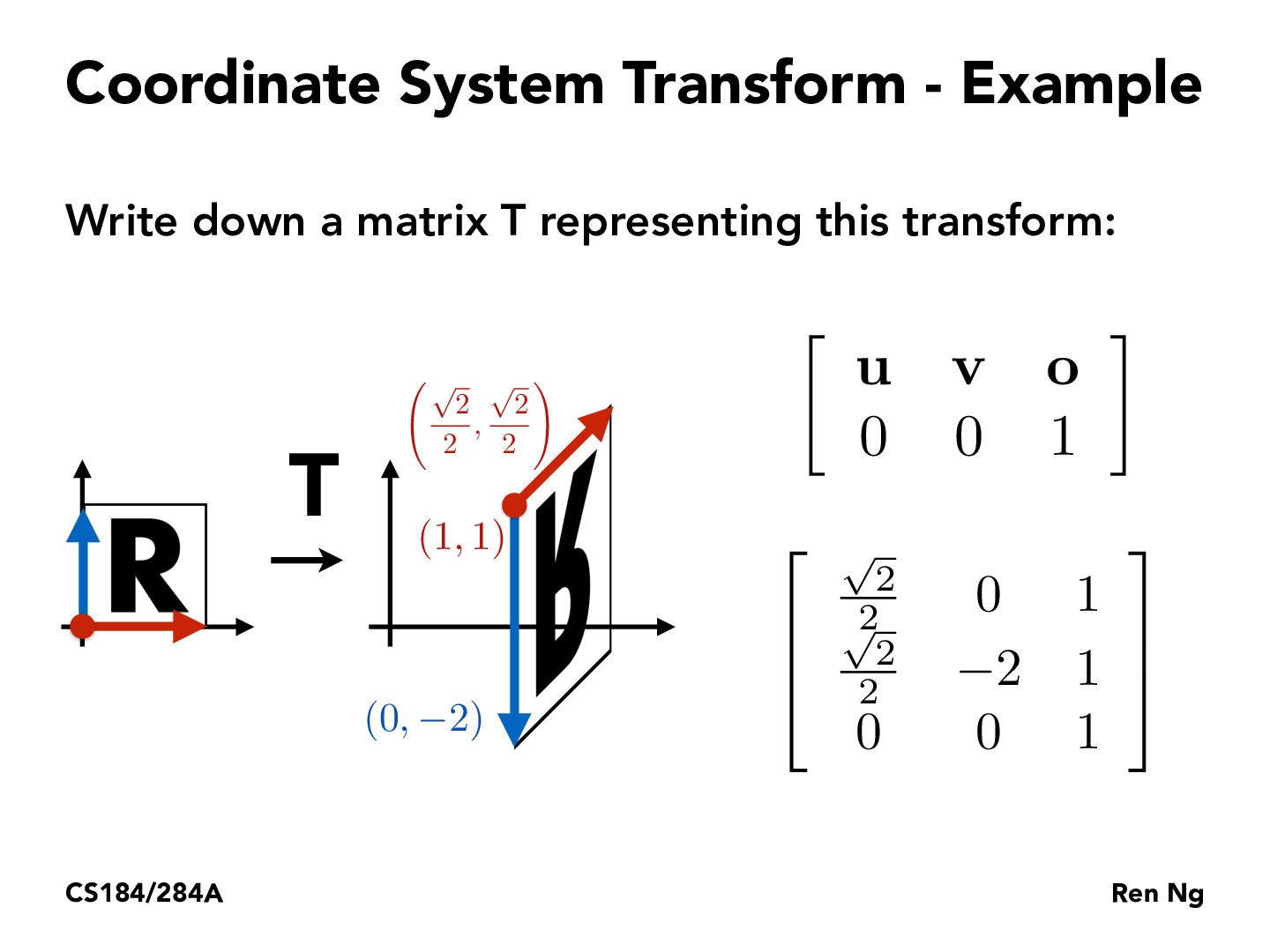Lecture 4: Transforms (40)sheaconlon

This example seems to work like the image alignment homework problems that some of us might remember from 16A. The two vectors define three points and three points are needed to uniquely recover the transformation.john-b-yang

Does it matter which vectors we assign to be "u" and "v"? In other words, if I switched the coordinates for the two so that the matrix looked like [[0, sqrt(2)/2, 1], [-2, sqrt(2)/2, 1]...], would that matter? My guess is that it does.

You must be enrolled in the course to comment